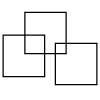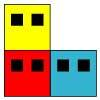#### You may also like### Four Triangles Puzzle

Cut four triangles from a square as shown in the picture. How many different shapes can you make by fitting the four triangles back together?### Three Squares

What is the greatest number of squares you can make by overlapping three squares?### A City of Towers

In this town, houses are built with one room for each person. There are some families of seven people living in the town. In how many different ways can they build their houses?

# Start Cube Drilling

##### Age 5 to 7Challenge Level

We had a few solutions come in from pupils around the world showing how they came to their answers. Firstly, Lesley from Kings Road Primary School in England, wrote;

16. When I drill my holes, I drill through three cubes at a time through each corner. When my friend drills through the top, he only drills one new cube at each corner because I have already drilled the rest.

Here is a solution by Louis, Katherine, Angus, William, Isobel and Harrison from St. Aiden's School England:

We started by making a cube from 27 Unifix cubes and we checked that it really was a 3 x 3 x 3 cube. We then imagined that we drilled a hole through the cube from the front face into each of the four corner cubes,  all the way to the back, marking the cubes with the holes in with bits of blutak.  12 of the cubes now had a hole in them. That is because on the front face we made 4 holes and each one went through 3 cubes until it got to the back. Then we looked down from the top face and repeated this and we marked all the 12 cubes with blutak. So we had made 24 holes in the cubes. But we found that some of the cubes had been marked twice and these were the corner cubes. Because a cube has 8 corner cubes, that meant we had to subtract 8 so we now had 24 - 8 = 16 cubes with holes in them.

We then thought about what would happen if we had a cube that was  4 x 4 x 4. This would have 64 small cubes. Again we started by drilling from the front and made 4 holes and this time they went through 4 cubes each so there were 4 x 4 = 16 cubes with holes in. If we did the same from the top there would be another 16 cubes with holes in so that is 32 altogether. But the corner cubes have 2 holes so we need to subtract 8 again so the total number of cubes is 32 - 8 = 24.

We think that whatever number you choose for making your cube (eg 3 x 3 x 3, or 4 x 4 x 4), you can do the same thing. You multiply the number by 4, then double it (front face and top face) and then subtract 8 (for the corners that have 2 holes) and that is the total number of holes you have drilled.

Thank you for that thorough explanation

Then Grace from Ohaupo Primary School in New Zealand sent in this:

I made a cube out of blocks and tallied up the bottom rows first, I got an answer of 6. Then there were the top rows, I also got an answer of 6 then. Then I had to drill in the friend's view.  I added those up and got an answer of four. I then added them to my answer of twelve. The answer I got overall was 16. So 11 cubes were not drilled.

Rik from Gdgoenka World School in India sent in the following:

The answer is 16, as we need to drill to the back first we drill 12 cubes, 3 for each back multiplied by 4 corners and we get 12. Then when your friend drills from up only 4 cubes are drilled. As 12 + 4 = 16 the answer is 16.

Finally Amran From Prioslee Primary Academy England:

So I imagined a 3 by 3 by 3 cube and thought if you drilled four holes and then another four holes you would add 4 + 4 which then equals 8 meaning there were 8 holes drilled in from the front and altogether looking from the front and the back there would be 16 holes.

Thank you all for these submissions. If you are looking at these with the idea of exploring the challenge further you should find their comments useful.